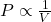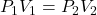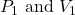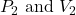g Select True or False: Boyle’s Law states that the volume of a fixed amount of gas maintained at constant temperature is directly proportio

Question

g Select True or False: Boyle’s Law states that the volume of a fixed amount of gas maintained at constant temperature is directly proportional to the gas pressure

in progress 0
6 months 2021-07-15T19:29:39+00:00 1 Answers 106 views 0

1. Answer: The given statement is False.

Explanation:

Boyle’s law states that pressure is inversely proportional to the volume of the gas at constant temperature and the number of moles.

Mathematically,(At constant temperature and number of moles)

ORwhere,are the initial pressure and volume of the gasare the final pressure and volume of the gas

Hence, the given statement is False.